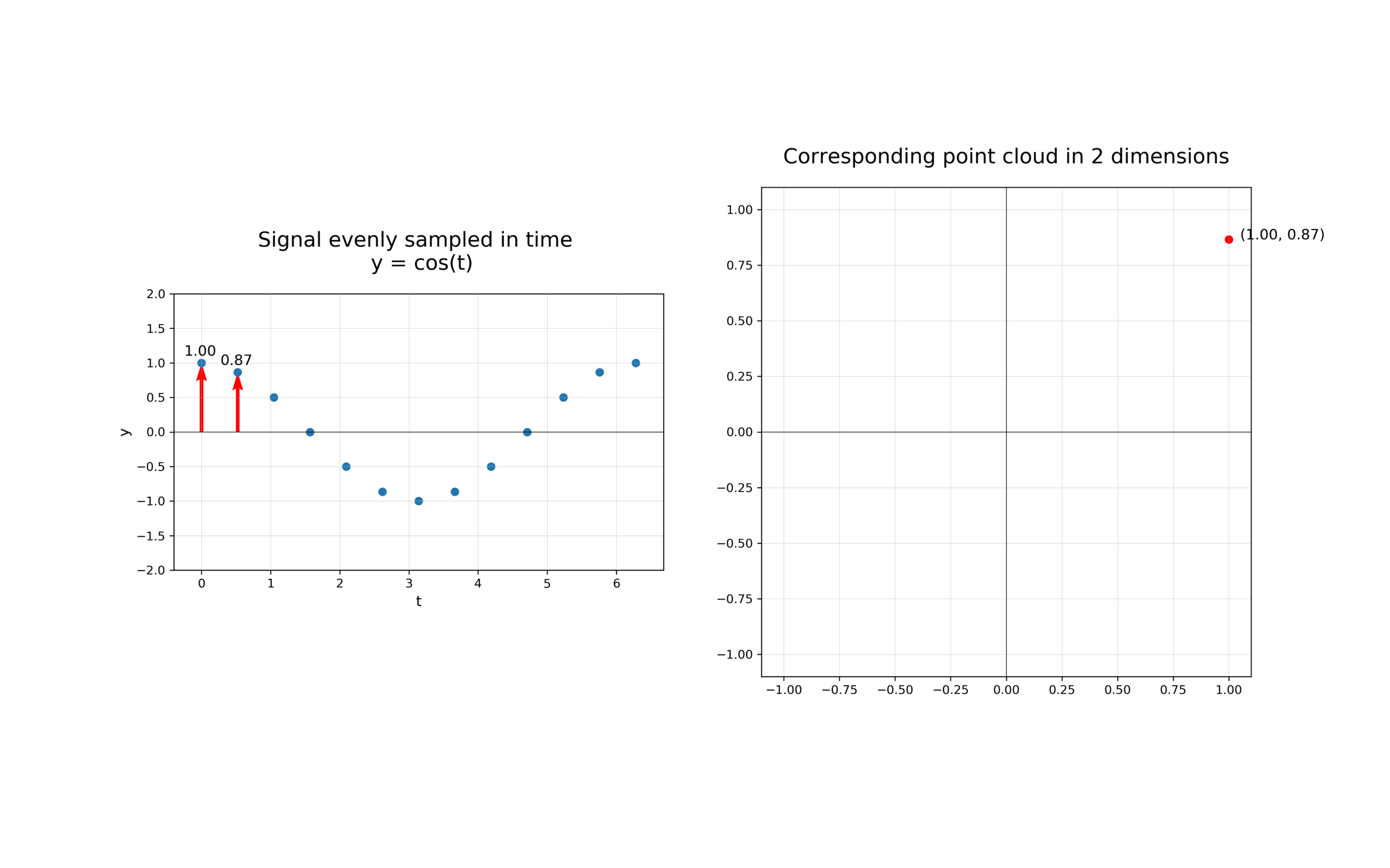# Topology of time series¶

This notebook explores how giotto-tda can be used to gain insights from time-varying data by using ideas from from dynamical systems and persistent homology.

If you are looking at a static version of this notebook and would like to run its contents, head over to GitHub and download the source.

## From time series to time delay embeddings¶

The first step in analysing the topology of time series is to construct a time delay embedding or Takens embedding, named after Floris Takens who pioneered its use in the study of dynamical systems. A time delay embedding can be thought of as sliding a “window” of fixed size over a signal, with each window represented as a point in a (possibly) higher-dimensional space. A simple example is shown in the animation below, where pairs of points in a 1-dimensional signal are mapped to coordinates in a 2-dimensional embedding space.A 2-dimensional time delay embedding

More formally, given a time series $$f(t)$$, one can extract a sequence of vectors of the form $$f_i = [f(t_i), f(t_i + 2 \tau), \ldots, f(t_i + (d-1) \tau)] \in \mathbb{R}^{d}$$, where $$d$$ is the embedding dimension and $$\tau$$ is the time delay. The quantity $$(d-1)\tau$$ is known as the “window size” and the difference between $$t_{i+1}$$ and $$t_i$$ is called the stride. In other words, the time delay embedding of $$f$$ with parameters $$(d,\tau)$$ is the function

$\begin{split}TD_{d,\tau} f : \mathbb{R} \to \mathbb{R}^{d}\,, \qquad t \to \begin{bmatrix} f(t) \\ f(t + \tau) \\ f(t + 2\tau) \\ \vdots \\ f(t + (d-1)\tau) \end{bmatrix}\end{split}$

and the main idea we will explore in this notebook is that if $$f$$ has a non-trivial recurrent structure, then the image of $$TD_{d,\tau}f$$ will have non-trivial topology for appropriate choices of $$(d, \tau)$$.

## A periodic example¶

As a warm-up, recall that a function is periodic with period $$T > 0$$ if $$f(t + T) = f(t)$$ for all $$t \in \mathbb{R}$$. For example, consider the function $$f(t) = \cos(5 t)$$ which can be visualised as follows:

import numpy as np
import plotly.graph_objects as go

x_periodic = np.linspace(0, 10, 1000)
y_periodic = np.cos(5 * x_periodic)

fig = go.Figure(data=go.Scatter(x=x_periodic, y=y_periodic))
fig.update_layout(xaxis_title="Timestamp", yaxis_title="Amplitude")
fig.show()